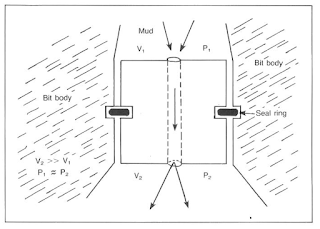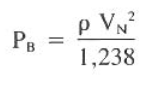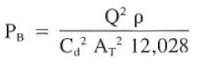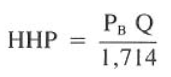# DRILL BIT PRESSURE DROP CALCULATION WITH MANY ONLINE CALCULATORS

## Introduction To Drilling Hydraulics Calculations

### Drill Bit Pressure Drop Calculation

Calculation of both hydraulic horsepower and impact force are mainly depend on calculating pressure drop across the bit. A major percentage of normal circulating pressures is lost by pumping through the nozzles when jet bits are used. The pressure loss is not frictional pressures but rather acceleration forces. Assumptions generally made areFig.1 : Bit Jet Flow
1) the changes in pressure resulting from a change in elevation are negligible.

2) the upstream velocity (V.) is negligible compared to downstream (as below figure).

Based on these assumptions, bit pressure drop is calculated according to below Equation:Solving for nozzle velocity, VN:

Laboratory studies show the pressure drop through the nozzle does not correspond precisely according to Eqs. above. As a result, a discharge coefficient factor, Cd is applied to make laboratory results correspond to theoretical pressure drops. The discharge coefficient will vary, depending on nozzle type and size, A value of 0.95 is representative, however, for most field situations.

Applying Cd above equation becomes:EQ.1
The velocity in the nozzles is equal to the flow volume divided by the area, or V = Q/A

Converting to common field units, the VN becomes as follows:EQ.2
Where:
AT = total nozzle area, in^2
Q = flow rate, gal/min
VN = nozzle velocity, ft/sec

Using Eq. 1&2 And solving for PB:EQ.3

### Drill Bit Hydraulic Horsepower & Impact Force Calculation

#### Hydraulic Horsepower at the drill bit

A measure of the energy per unit of time that is being expended across the bit nozzles. It is commonly calculated with the equation HHP=P*Q/1714, where P stands for pressure drop in pounds per square in., Q stands for flow rate in gallons per minute, and 1714 is a conversion factor necessary to yield HHP in terms of horsepower. Bit manufacturers often recommend that fluid hydraulics energy across the bit nozzles be in a particular HHP range, for example 2.0 to 7.0 HHP, to ensure adequate bit tooth and bottom-of-hole cleaning (the minimum HHP) and to avoid premature erosion of the bit itself (the maximum HHP).EQ.4

#### Impact Force Calculations at the drill bit

Impact pressure which affect impact force is the momentum change of the drilling fluid distributed over the impacted area at the hole bottom. This pressure, acting downward and to the sides, attempts to overcome chip hold-down pressure and remove cutting from beneath the bit. pressure and remove cutting from beneath the bit.

In drilling Hydraulics, Impact Force can be calculated through this equation.EQ.5

### Example On How To Calculate bit pressure drop, Hydraulic Horse Power And Impact Force

Calculate the drill bit pressure drop, hydraulic horsepower and impact force for the following set of conditions:

Mud weight = 14.5 Ib/gal
Flow rate = 300 gal/min
Jet sizes = three 12/32 in. jets

Solution:

1. Determine the nozzle area, AT:
AT = 3 π /4 (d)^2 = 0.331 in^2

2. Eq.3 is used to calculate the pressure drop at the bit:
PB (300)^ 2 x 14.5 ( 0.95^2 0.331 ^2 12028) = 1097 psi

3. Calculate the hydraulic horsepower expended at the bit from Eq.4
HHP = (1097 psi 300 gal/min) 1714 = 192 hp

4. The hydraulic impact force due to pressure loss through the drilling bit is computed from Eq.5
Fi = 0.01823 (0.95) (300) ((14.5)(1,097))^(1/2) = 654 Ib